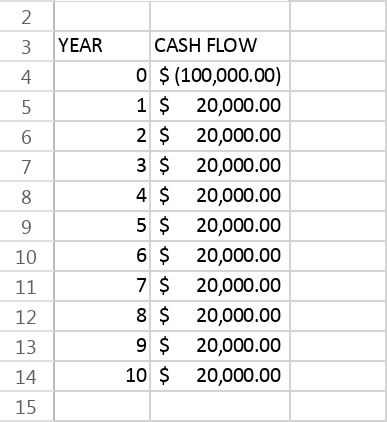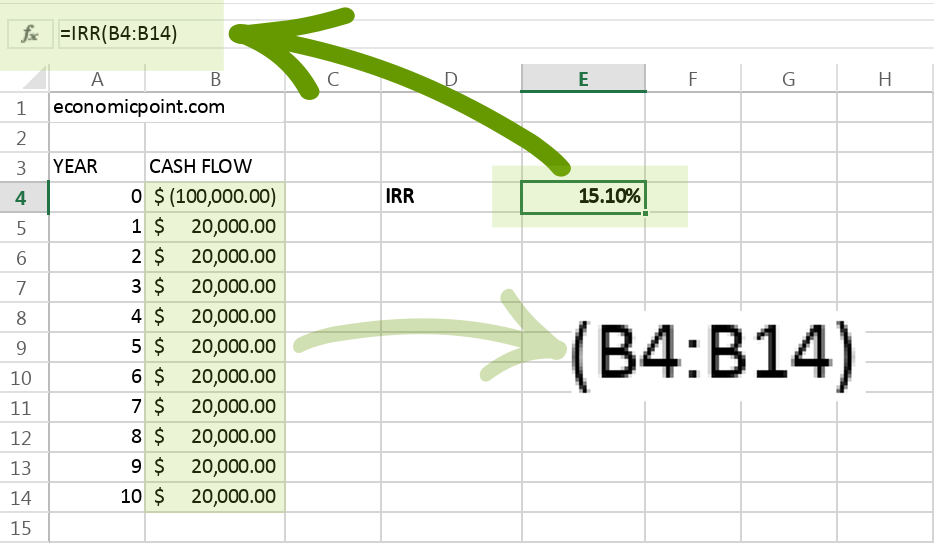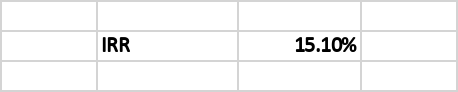# IRR calculation in Excel

This article teaches you how to calculate the IRR (Internal Return Rate) using Excel. The Excel function to calculate the IRR is “IRR” and it’s really simple, since it only takes the range that contains the flow of funds, including the initial investment.

The IRR, or Internal Rate of Return, is the interest rate that makes the present value of a flow of funds equal to zero. The present value of a cash flow is the current worth of it. To know the current value, you must use a discount rate. The IRR calculation includes all positive and negative flows, it includes the initial investment.

To know if an investment project is desirable, we must compare the IRR with the rate of return of the best available investment alternative. If the IRR is greater than the rate of the best alternative, the project is desirable.

STEP 1: Know your Cash Flow

The first step to calculate the IRR using Excel is to input all the cash flows of the investment project.

Future cash flows are usually estimated using a model.STEP 3: Enter the IRR Excel Formula

The IRR formula requires only one arguments, the range that containing the cash flows, including the initial investment.

## Excel Formula:

`=IRR(B4:B14)`

Where:
B4:B14: range containing the future cash flowsPress Enter and Excel will calculate the IRR.